x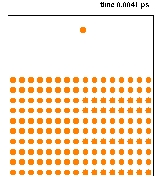Molecular dynamicsOverview

Molecular dynamics
Dynamics (mechanics)
In the field of physics, the study of the causes of motion and changes in motion is dynamics. In other words the study of forces and why objects are in motion. Dynamics includes the study of the effect of torques on motion...

(MD) is a computer simulation
Computer simulation
A computer simulation, a computer model, or a computational model is a computer program, or network of computers, that attempts to simulate an abstract model of a particular system...

of physical movements
Motion (physics)
In physics, motion is a change in position of an object with respect to time. Change in action is the result of an unbalanced force. Motion is typically described in terms of velocity, acceleration, displacement and time . An object's velocity cannot change unless it is acted upon by a force, as...

of atoms and molecules. The atoms and molecules are allowed to interact for a period of time, giving a view of the motion of the atoms. In the most common version, the trajectories of molecules and atoms are determined by numerically solving
Numerical integration
In numerical analysis, numerical integration constitutes a broad family of algorithms for calculating the numerical value of a definite integral, and by extension, the term is also sometimes used to describe the numerical solution of differential equations. This article focuses on calculation of...

the Newton's equations of motion
Newton's laws of motion
Newton's laws of motion are three physical laws that form the basis for classical mechanics. They describe the relationship between the forces acting on a body and its motion due to those forces...

for a system of interacting particles, where forces between the particles and potential energy
Potential energy
In physics, potential energy is the energy stored in a body or in a system due to its position in a force field or due to its configuration. The SI unit of measure for energy and work is the Joule...

are defined by molecular mechanics
Molecular mechanics
Molecular mechanics uses Newtonian mechanics to model molecular systems. The potential energy of all systems in molecular mechanics is calculated using force fields...

force field
Force field (chemistry)
In the context of molecular modeling, a force field refers to the form and parameters of mathematical functions used to describe the potential energy of a system of particles . Force field functions and parameter sets are derived from both experimental work and high-level quantum mechanical...

s.Discussions1Site CO2 vs 3SIte CO2Encyclopedia
Molecular dynamics
Dynamics (mechanics)
In the field of physics, the study of the causes of motion and changes in motion is dynamics. In other words the study of forces and why objects are in motion. Dynamics includes the study of the effect of torques on motion...

(MD) is a computer simulation
Computer simulation
A computer simulation, a computer model, or a computational model is a computer program, or network of computers, that attempts to simulate an abstract model of a particular system...

of physical movements
Motion (physics)
In physics, motion is a change in position of an object with respect to time. Change in action is the result of an unbalanced force. Motion is typically described in terms of velocity, acceleration, displacement and time . An object's velocity cannot change unless it is acted upon by a force, as...

of atoms and molecules. The atoms and molecules are allowed to interact for a period of time, giving a view of the motion of the atoms. In the most common version, the trajectories of molecules and atoms are determined by numerically solving
Numerical integration
In numerical analysis, numerical integration constitutes a broad family of algorithms for calculating the numerical value of a definite integral, and by extension, the term is also sometimes used to describe the numerical solution of differential equations. This article focuses on calculation of...

the Newton's equations of motion
Newton's laws of motion
Newton's laws of motion are three physical laws that form the basis for classical mechanics. They describe the relationship between the forces acting on a body and its motion due to those forces...

for a system of interacting particles, where forces between the particles and potential energy
Potential energy
In physics, potential energy is the energy stored in a body or in a system due to its position in a force field or due to its configuration. The SI unit of measure for energy and work is the Joule...

are defined by molecular mechanics
Molecular mechanics
Molecular mechanics uses Newtonian mechanics to model molecular systems. The potential energy of all systems in molecular mechanics is calculated using force fields...

force field
Force field (chemistry)
In the context of molecular modeling, a force field refers to the form and parameters of mathematical functions used to describe the potential energy of a system of particles . Force field functions and parameter sets are derived from both experimental work and high-level quantum mechanical...

s. The method was originally conceived within theoretical physics in the late 1950s and early 1960s
, but is applied today mostly in materials science
Materials science
Materials science is an interdisciplinary field applying the properties of matter to various areas of science and engineering. This scientific field investigates the relationship between the structure of materials at atomic or molecular scales and their macroscopic properties. It incorporates...

and the modeling of biomolecule
Biomolecule
A biomolecule is any molecule that is produced by a living organism, including large polymeric molecules such as proteins, polysaccharides, lipids, and nucleic acids as well as small molecules such as primary metabolites, secondary metabolites, and natural products...

s.

Because molecular systems consist of a vast number of particles, it is impossible to find the properties of such complex systems
Complex systems
Complex systems present problems in mathematical modelling.The equations from which complex system models are developed generally derive from statistical physics, information theory and non-linear dynamics, and represent organized but unpredictable behaviors of systems of nature that are considered...

analytically; MD simulation circumvents this problem by using numerical
Numerical analysis
Numerical analysis is the study of algorithms that use numerical approximation for the problems of mathematical analysis ....

methods. However, long MD simulations are mathematically ill-conditioned
Condition number
In the field of numerical analysis, the condition number of a function with respect to an argument measures the asymptotically worst case of how much the function can change in proportion to small changes in the argument...

, generating cumulative errors in numerical integration
Numerical integration
In numerical analysis, numerical integration constitutes a broad family of algorithms for calculating the numerical value of a definite integral, and by extension, the term is also sometimes used to describe the numerical solution of differential equations. This article focuses on calculation of...

that can be minimized with proper selection of algorithms and parameters, but not eliminated entirely.

The results of molecular dynamics simulations may be used to determine macroscopic thermodynamic properties of the system based on the ergodic hypothesis
Ergodic hypothesis
In physics and thermodynamics, the ergodic hypothesis says that, over long periods of time, the time spent by a particle in some region of the phase space of microstates with the same energy is proportional to the volume of this region, i.e., that all accessible microstates are equiprobable over a...

: the statistical ensemble averages are equal to time averages of the system. MD has also been termed "statistical mechanics by numbers" and "Laplace's vision of Newtonian mechanics" of predicting the future by animating nature's forces and allowing insight into molecular motion on an atomic scale.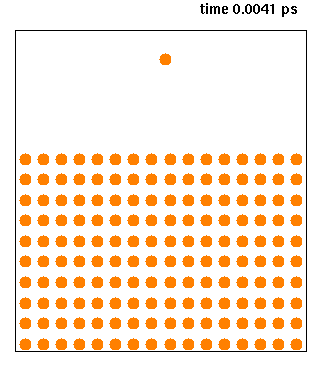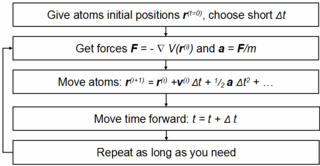## History

Before it became possible to simulate molecular dynamics with computers, some undertook the hard work of trying it with physical models such as macroscopic spheres. The idea was to arrange them to replicate the properties of a liquid. J.D. Bernal said, in 1962: "... I took a number of rubber balls and stuck them together with rods of a selection of different lengths ranging from 2.75 to 4 inches. I tried to do this in the first place as casually as possible, working in my own office, being interrupted every five minutes or so and not remembering what I had done before the interruption." Fortunately, now computers keep track of bonds during a simulation.

## Areas of Application

There is a significant difference between the focus and methods used by chemists and physicists, and this is reflected in differences in the jargon used by the different fields. In chemistry and biophysics, the interaction between the particles is either described by a "force field
Force field (chemistry)
In the context of molecular modeling, a force field refers to the form and parameters of mathematical functions used to describe the potential energy of a system of particles . Force field functions and parameter sets are derived from both experimental work and high-level quantum mechanical...

" (classical MD), a quantum chemical model
Quantum chemistry
Quantum chemistry is a branch of chemistry whose primary focus is the application of quantum mechanics in physical models and experiments of chemical systems...

, or a mix between the two. These terms are not used in physics, where the interactions are usually described by the name of the theory or approximation being used and called the potential energy, or just the "potential".

Beginning in theoretical physics
Physics
Physics is a natural science that involves the study of matter and its motion through spacetime, along with related concepts such as energy and force. More broadly, it is the general analysis of nature, conducted in order to understand how the universe behaves.Physics is one of the oldest academic...

, the method of MD gained popularity in materials science
Materials science
Materials science is an interdisciplinary field applying the properties of matter to various areas of science and engineering. This scientific field investigates the relationship between the structure of materials at atomic or molecular scales and their macroscopic properties. It incorporates...

and since the 1970s also in biochemistry
Biochemistry
Biochemistry, sometimes called biological chemistry, is the study of chemical processes in living organisms, including, but not limited to, living matter. Biochemistry governs all living organisms and living processes...

and biophysics
Biophysics
Biophysics is an interdisciplinary science that uses the methods of physical science to study biological systems. Studies included under the branches of biophysics span all levels of biological organization, from the molecular scale to whole organisms and ecosystems...

. In chemistry, MD serves as an important tool in protein
Protein
Proteins are biochemical compounds consisting of one or more polypeptides typically folded into a globular or fibrous form, facilitating a biological function. A polypeptide is a single linear polymer chain of amino acids bonded together by peptide bonds between the carboxyl and amino groups of...

structure determination and refinement using experimental tools such as X-ray crystallography
X-ray crystallography
X-ray crystallography is a method of determining the arrangement of atoms within a crystal, in which a beam of X-rays strikes a crystal and causes the beam of light to spread into many specific directions. From the angles and intensities of these diffracted beams, a crystallographer can produce a...

and NMR
NMR
NMR may refer to:Applications of Nuclear Magnetic Resonance:* Nuclear magnetic resonance* NMR spectroscopy* Solid-state nuclear magnetic resonance* Protein nuclear magnetic resonance spectroscopy* Proton NMR* Carbon-13 NMR...

. It has also been applied with limited success as a method of refining protein structure prediction
Protein structure prediction
Protein structure prediction is the prediction of the three-dimensional structure of a protein from its amino acid sequence — that is, the prediction of its secondary, tertiary, and quaternary structure from its primary structure. Structure prediction is fundamentally different from the inverse...

s. In physics, MD is used to examine the dynamics of atomic-level phenomena that cannot be observed directly, such as thin film growth and ion-subplantation. It is also used to examine the physical properties of nanotechnological
Nanotechnology
Nanotechnology is the study of manipulating matter on an atomic and molecular scale. Generally, nanotechnology deals with developing materials, devices, or other structures possessing at least one dimension sized from 1 to 100 nanometres...

devices that have not or cannot yet be created.

In applied mathematics and theoretical physics, molecular dynamics is a part of the research realm of dynamical systems, ergodic theory
Ergodic theory
Ergodic theory is a branch of mathematics that studies dynamical systems with an invariant measure and related problems. Its initial development was motivated by problems of statistical physics....

, Atomic, molecular, and optical physics
Atomic, molecular, and optical physics
Atomic, molecular, and optical physics is the study of matter-matter and light-matter interactions on the scale of single atoms or structures containing a few atoms. The three areas are grouped together because of their interrelationships, the similarity of methods used, and the commonality of the...

and statistical mechanics
Statistical mechanics
Statistical mechanics or statistical thermodynamicsThe terms statistical mechanics and statistical thermodynamics are used interchangeably...

in general. The concepts of energy conservation and molecular entropy come from thermodynamics
Thermodynamics
Thermodynamics is a physical science that studies the effects on material bodies, and on radiation in regions of space, of transfer of heat and of work done on or by the bodies or radiation...

. Some techniques to calculate conformational entropy
Conformational entropy
Conformational entropy is the entropy associated with the physical arrangement of a polymer chain that assumes a compact or globular state in solution. The concept is most commonly applied to biological macromolecules such as proteins and RNA, but can also be used for polysaccharides and other...

such as principal components analysis
Principal components analysis
Principal component analysis is a mathematical procedure that uses an orthogonal transformation to convert a set of observations of possibly correlated variables into a set of values of uncorrelated variables called principal components. The number of principal components is less than or equal to...

come from information theory
Entropy in thermodynamics and information theory
There are close parallels between the mathematical expressions for the thermodynamic entropy, usually denoted by S, of a physical system in the statistical thermodynamics established by Ludwig Boltzmann and J. Willard Gibbs in the 1870s; and the information-theoretic entropy, usually expressed as...

. Mathematical techniques such as the transfer operator
Transfer operator
In mathematics, the transfer operator encodes information about an iterated map and is frequently used to study the behavior of dynamical systems, statistical mechanics, quantum chaos and fractals...

become applicable when MD is seen as a Markov chain
Markov chain
A Markov chain, named after Andrey Markov, is a mathematical system that undergoes transitions from one state to another, between a finite or countable number of possible states. It is a random process characterized as memoryless: the next state depends only on the current state and not on the...

. Also, there is a large community of mathematicians working on volume preserving, symplectic integrator
Symplectic integrator
In mathematics, a symplectic integrator is a numerical integration scheme for a specific group of differential equations related to classical mechanics and symplectic geometry. Symplectic integrators form the subclass of geometric integrators which, by definition, are canonical transformations...

s for more computationally efficient MD simulations.

MD can also be seen as a special case of the discrete element method
Discrete element method
A discrete element method , also called a distinct element method is any of family of numerical methods for computing the motion of a large number of particles of micrometre-scale size and above...

(DEM) in which the particles have spherical shape (e.g. with the size of their van der Waals radii
The van der Waals radius, r, of an atom is the radius of an imaginary hard sphere which can be used to model the atom for many purposes. It is named after Johannes Diderik van der Waals, winner of the 1910 Nobel Prize in Physics, as he was the first to recognise that atoms had a finite size and to...

.) Some authors in the DEM community employ the term MD rather loosely, even when their simulations do not model actual molecules.

### Steered Molecular Dynamics (SMD)

Steered molecular dynamics (SMD) simulations, or Force Probe Simulations, apply forces to a protein in order to manipulate its structure by pulling it along desired degrees of freedom. These experiments can be used to reveal structural changes in a protein at the atomic level. SMD is often used to simulate events such as mechanical unfolding or stretching.

There are two typical protocols of SMD: one in which pulling velocity is held constant and one in which applied force is constant. Typically, part of the studied system (eg. An atom in a protein) is restrained by a harmonic potential. Forces are then applied to specific atoms at either a constant velocity or a constant force. Umbrella Sampling
Umbrella sampling
Umbrella sampling is a technique in computational physics and chemistry, used to improve sampling of a system where ergodicity is hindered by the form of the system's energy landscape. It was first suggested by Torrie and Valleau in 1977...

is used to move the system along the desired reaction coordinate by varying, for example, the forces, distances, and angles manipulated in the simulation. Through umbrella sampling, all of the system's configurations—both high-energy and low-energy—are adequately sampled. Then, each configuration's change in free energy can be calculated as the potential mean force. A popular method of computing PMF is through the weighted histogram analysis method (WHAM), which analyzes a series of umbrella sampling simulations.

## Design Constraints

Design of a molecular dynamics simulation should account for the available computational power. Simulation size (n=number of particles), timestep and total time duration must be selected so that the calculation can finish within a reasonable time period. However, the simulations should be long enough to be relevant to the time scales of the natural processes being studied. To make statistically valid conclusions from the simulations, the time span simulated should match the kinetics of the natural process. Otherwise, it is analogous to making conclusions about how a human walks from less than one footstep. Most scientific publications about the dynamics of proteins and DNA use data from simulations spanning nanoseconds (10−9 s) to microseconds (10−6 s). To obtain these simulations, several CPU-days to CPU-years are needed. Parallel algorithms allow the load to be distributed among CPUs; an example is the spatial or force decomposition algorithm http://www.sandia.gov/~sjplimp/md.html.

During a classical MD simulation, the most CPU intensive task is the evaluation of the potential (force field
Force field (chemistry)
In the context of molecular modeling, a force field refers to the form and parameters of mathematical functions used to describe the potential energy of a system of particles . Force field functions and parameter sets are derived from both experimental work and high-level quantum mechanical...

) as a function of the particles' internal coordinates. Within that energy evaluation, the most expensive one is the non-bonded or non-covalent part. In Big O notation
Big O notation
In mathematics, big O notation is used to describe the limiting behavior of a function when the argument tends towards a particular value or infinity, usually in terms of simpler functions. It is a member of a larger family of notations that is called Landau notation, Bachmann-Landau notation, or...

, common molecular dynamics simulations scale
Analysis of algorithms
To analyze an algorithm is to determine the amount of resources necessary to execute it. Most algorithms are designed to work with inputs of arbitrary length...

by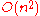if all pair-wise electrostatic and van der Waals interactions must be accounted for explicitly. This computational cost can be reduced by employing electrostatics methods such as Particle Mesh Ewald
Ewald summation
Ewald summation, named after Paul Peter Ewald, is a method for computing the interaction energies of periodic systems , particularly electrostatic energies. Ewald summation is a special case of the Poisson summation formula, replacing the summation of interaction energies in real space with an...

(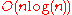), P3M
P3M
Particle-Particle-Particle Mesh is a Fourier-based Ewald summation method to calculate potentials in N-body simulations.The potential could be the electrostatic potential among N point charges i.e. molecular dynamics, the gravitational potential among N gas particles in e.g. smoothed particle...

or good spherical cutoff techniques (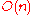).

Another factor that impacts total CPU time required by a simulation is the size of the integration timestep. This is the time length between evaluations of the potential. The timestep must be chosen small enough to avoid discretization
Discretization
In mathematics, discretization concerns the process of transferring continuous models and equations into discrete counterparts. This process is usually carried out as a first step toward making them suitable for numerical evaluation and implementation on digital computers...

errors (i.e. smaller than the fastest vibrational frequency in the system). Typical timesteps for classical MD are in the order of 1 femtosecond (10−15 s). This value may be extended by using algorithms such as SHAKE
Constraint algorithm
In mechanics, a constraint algorithm is a method for satisfying constraints for bodies that obey Newton's equations of motion. There are three basic approaches to satisfying such constraints: choosing novel unconstrained coordinates , introducing explicit constraint forces, and minimizing...

, which fix the vibrations of the fastest atoms (e.g. hydrogens) into place. Multiple time scale methods have also been developed, which allow for extended times between updates of slower long-range forces.

For simulating molecules in a solvent, a choice should be made between explicit solvent
Water model
In computational chemistry, classical water models are used for the simulation of water clusters, liquid water, and aqueous solutions with explicit solvent. These models use the approximations of molecular mechanics...

and implicit solvent. Explicit solvent particles (such as the TIP3P, SPC/E and SPC-f
Flexible SPC water model
The Flexible Simple Point Charge water model is a re-parametrization of the three-site SPC water model. The SPC model is rigid, whilst the flexible SPC model is flexible. In the model of Toukan and Rahman, the O-H stretching is made anharmonic and thus the dynamical behavior is well described...

water models) must be calculated expensively by the force field, while implicit solvents use a mean-field approach. Using an explicit solvent is computationally expensive, requiring inclusion of roughly ten times more particles in the simulation. But the granularity and viscosity of explicit solvent is essential to reproduce certain properties of the solute molecules. This is especially important to reproduce kinetics
Chemical kinetics
Chemical kinetics, also known as reaction kinetics, is the study of rates of chemical processes. Chemical kinetics includes investigations of how different experimental conditions can influence the speed of a chemical reaction and yield information about the reaction's mechanism and transition...

.

In all kinds of molecular dynamics simulations, the simulation box size must be large enough to avoid boundary condition artifacts. Boundary conditions are often treated by choosing fixed values at the edges (which may cause artifacts), or by employing periodic boundary conditions
Periodic boundary conditions
In mathematical models and computer simulations, periodic boundary conditions are a set of boundary conditions that are often used to simulate a large system by modelling a small part that is far from its edge...

in which one side of the simulation loops back to the opposite side, mimicking a bulk phase.

### Microcanonical ensemble (NVE)

In the microcanonical, or NVE ensemble, the system is isolated from changes in moles (N), volume (V) and energy (E). It corresponds to an adiabatic process
In thermodynamics, an adiabatic process or an isocaloric process is a thermodynamic process in which the net heat transfer to or from the working fluid is zero. Such a process can occur if the container of the system has thermally-insulated walls or the process happens in an extremely short time,...

with no heat exchange. A microcanonical molecular dynamics trajectory may be seen as an exchange of potential and kinetic energy, with total energy being conserved. For a system of N particles with coordinates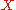and velocities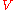, the following pair of first order differential equations may be written in Newton's notation
Newton's notation for differentiation
Newton's notation for differentiation, or dot notation, uses a dot placed over a function name to denote the time derivative of that function. Newton referred to this as a fluxion.Isaac Newton's notation is mainly used in mechanics...

as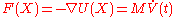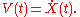The potential energy function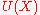of the system is a function of the particle coordinates. It is referred to simply as the "potential" in Physics, or the "force field" in Chemistry. The first equation comes from Newton's laws
Newton's laws of motion
Newton's laws of motion are three physical laws that form the basis for classical mechanics. They describe the relationship between the forces acting on a body and its motion due to those forces...

; the force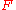acting on each particle in the system can be calculated as the negative gradient of.

For every timestep, each particle's positionand velocitymay be integrated with a symplectic method
Symplectic integrator
In mathematics, a symplectic integrator is a numerical integration scheme for a specific group of differential equations related to classical mechanics and symplectic geometry. Symplectic integrators form the subclass of geometric integrators which, by definition, are canonical transformations...

such as Verlet
Verlet integration
Verlet integration is a numerical method used to integrate Newton's equations of motion. It is frequently used to calculate trajectories of particles in molecular dynamics simulations and computer graphics...

. The time evolution ofandis called a trajectory. Given the initial positions (e.g. from theoretical knowledge) and velocities (e.g. randomized Gaussian), we can calculate all future (or past) positions and velocities.

One frequent source of confusion is the meaning of temperature
Temperature
Temperature is a physical property of matter that quantitatively expresses the common notions of hot and cold. Objects of low temperature are cold, while various degrees of higher temperatures are referred to as warm or hot...

in MD. Commonly we have experience with macroscopic temperatures, which involve a huge number of particles. But temperature is a statistical quantity. If there is a large enough number of atoms, statistical temperature can be estimated from the instantaneous temperature, which is found by equating the kinetic energy of the system to nkBT/2 where n is the number of degrees of freedom of the system.

A temperature-related phenomenon arises due to the small number of atoms that are used in MD simulations. For example, consider simulating the growth of a copper film starting with a substrate containing 500 atoms and a deposition energy of 100 eV. In the real world, the 100 eV from the deposited atom would rapidly be transported through and shared among a large number of atoms (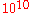or more) with no big change in temperature. When there are only 500 atoms, however, the substrate is almost immediately vaporized by the deposition. Something similar happens in biophysical simulations. The temperature of the system in NVE is naturally raised when macromolecules such as proteins undergo exothermic conformational changes and binding.

### Canonical ensemble (NVT)

In the canonical ensemble
Canonical ensemble
The canonical ensemble in statistical mechanics is a statistical ensemble representing a probability distribution of microscopic states of the system...

, moles (N), volume (V) and temperature (T) are conserved. It is also sometimes called constant temperature molecular dynamics (CTMD). In NVT, the energy of endothermic and exothermic processes is exchanged with a thermostat.

A variety of thermostat methods is available to add and remove energy from the boundaries of an MD system in a more or less realistic way, approximating the canonical ensemble
Canonical ensemble
The canonical ensemble in statistical mechanics is a statistical ensemble representing a probability distribution of microscopic states of the system...

. Popular techniques to control temperature include velocity rescaling, the Nosé-Hoover thermostat
Nosé-Hoover thermostat
The Nosé–Hoover thermostat is a deterministic method used in molecular dynamics to keep the temperature around an average. It was originally introduced by Nosé and developed further by Hoover...

, Nosé-Hoover chains, the Berendsen thermostat
Berendsen thermostat
The Berendsen thermostat is an algorithm to re-scale the velocities of particles in molecular dynamics simulations to control the simulation temperature.-Basic Description:In this scheme, the system is weakly coupled to a heat bath with some temperature...

and Langevin dynamics
Langevin dynamics
In physics, Langevin dynamics is an approach to the mathematical modeling of the dynamics of molecular systems, originally developed by the French physicist Paul Langevin...

. Note that the Berendsen thermostat might introduce the flying ice cube
Flying ice cube
In molecular dynamics simulations, the flying ice cube effect is a numerical integration artifact in which the energy of high-frequency fundamental modes is drained into low-frequency modes, particularly into zero-frequency motions such as overall translation and rotation of the system...

effect, which leads to unphysical translations and rotations of the simulated system.

It is not trivial to obtain a canonical distribution
Canonical ensemble
The canonical ensemble in statistical mechanics is a statistical ensemble representing a probability distribution of microscopic states of the system...

of conformations and velocities using these algorithms. How this depends on system size, thermostat choice, thermostat parameters, time step and integrator is the subject of many articles in the field.

### Isothermal-Isobaric (NPT) ensemble

In the isothermal-isobaric ensemble
Isothermal-isobaric ensemble
The isothermal–isobaric ensemble is a statistical mechanical ensemble that maintains constant temperature T \, and constant pressure P \, applied. It is also called the NpT-ensemble, where the number of particles N \, is also kept as a constant...

, moles (N), pressure (P) and temperature (T) are conserved. In addition to a thermostat, a barostat is needed. It corresponds most closely to laboratory conditions with a flask open to ambient temperature and pressure.

In the simulation of biological membranes, isotropic pressure control is not appropriate. For lipid bilayers, pressure control occurs under constant membrane area (NPAT) or constant surface tension "gamma" (NPγT).

### Generalized ensembles

The replica exchange method is a generalized ensemble. It was originally created to deal with the slow dynamics of disordered spin systems. It is also called parallel tempering. The replica exchange MD (REMD) formulation tries to overcome the multiple-minima problem by exchanging the temperature of non-interacting replicas of the system running at several temperatures.

## Potentials in MD simulations

A molecular dynamics simulation requires the definition of a potential function
Potential function
The term potential function may refer to:* A mathematical function whose values are a physical potential.* The class of functions known as harmonic functions, which are the topic of study in potential theory.* The potential function of a potential game....

, or a description of the terms by which the particles in the simulation will interact. In chemistry and biology this is usually referred to as a force field
Force field (chemistry)
In the context of molecular modeling, a force field refers to the form and parameters of mathematical functions used to describe the potential energy of a system of particles . Force field functions and parameter sets are derived from both experimental work and high-level quantum mechanical...

. Potentials may be defined at many levels of physical accuracy; those most commonly used in chemistry are based on molecular mechanics
Molecular mechanics
Molecular mechanics uses Newtonian mechanics to model molecular systems. The potential energy of all systems in molecular mechanics is calculated using force fields...

and embody a classical
Classical mechanics
In physics, classical mechanics is one of the two major sub-fields of mechanics, which is concerned with the set of physical laws describing the motion of bodies under the action of a system of forces...

treatment of particle-particle interactions that can reproduce structural and conformational change
Conformational change
A macromolecule is usually flexible and dynamic. It can change its shape in response to changes in its environment or other factors; each possible shape is called a conformation, and a transition between them is called a conformational change...

s but usually cannot reproduce chemical reaction
Chemical reaction
A chemical reaction is a process that leads to the transformation of one set of chemical substances to another. Chemical reactions can be either spontaneous, requiring no input of energy, or non-spontaneous, typically following the input of some type of energy, such as heat, light or electricity...

s.

The reduction from a fully quantum description to a classical potential entails two main approximations. The first one is the Born-Oppenheimer approximation
Born-Oppenheimer approximation
In quantum chemistry, the computation of the energy and wavefunction of an average-size molecule is a formidable task that is alleviated by the Born–Oppenheimer approximation, named after Max Born and J. Robert Oppenheimer. For instance the benzene molecule consists of 12 nuclei and 42...

, which states that the dynamics of electrons is so fast that they can be considered to react instantaneously to the motion of their nuclei. As a consequence, they may be treated separately. The second one treats the nuclei, which are much heavier than electrons, as point particles that follow classical Newtonian dynamics. In classical molecular dynamics the effect of the electrons is approximated as a single potential energy surface, usually representing the ground state. A quantum description is still used for the dynamics of the electrons when these approximations are made.

When finer levels of detail are required, potentials based on quantum mechanics
Quantum mechanics
Quantum mechanics, also known as quantum physics or quantum theory, is a branch of physics providing a mathematical description of much of the dual particle-like and wave-like behavior and interactions of energy and matter. It departs from classical mechanics primarily at the atomic and subatomic...

are used; some techniques attempt to create hybrid classical/quantum
QM/MM
The hybrid QM/MM approach is a molecular simulation method that combines the strength of both QM and MM calculations, thus allowing for the study of chemical processes in solution and in proteins. The QM/MM approach was introduced in the 1976 paper of Warshel and Levitt .An important advantage...

potentials where the bulk of the system is treated classically but a small region is treated as a quantum system, usually undergoing a chemical transformation.

### Empirical potentials

Empirical potentials used in chemistry are frequently called force fields, while those used in materials physics are called just empirical or analytical potentials.

Most force fields
Force field (chemistry)
In the context of molecular modeling, a force field refers to the form and parameters of mathematical functions used to describe the potential energy of a system of particles . Force field functions and parameter sets are derived from both experimental work and high-level quantum mechanical...

in chemistry are empirical and consist of a summation of bonded forces associated with chemical bond
Chemical bond
A chemical bond is an attraction between atoms that allows the formation of chemical substances that contain two or more atoms. The bond is caused by the electromagnetic force attraction between opposite charges, either between electrons and nuclei, or as the result of a dipole attraction...

s, bond angles, and bond dihedrals
Dihedral angle
In geometry, a dihedral or torsion angle is the angle between two planes.The dihedral angle of two planes can be seen by looking at the planes "edge on", i.e., along their line of intersection...

, and non-bonded forces associated with van der Waals force
Van der Waals force
In physical chemistry, the van der Waals force , named after Dutch scientist Johannes Diderik van der Waals, is the sum of the attractive or repulsive forces between molecules other than those due to covalent bonds or to the electrostatic interaction of ions with one another or with neutral...

s and electrostatic charge. Empirical potentials represent quantum-mechanical effects in a limited way through ad-hoc functional approximations. These potentials contain free parameters such as atomic charge, van der Waals
Van der Waals
-People:* Fransje van der Waals , Dutch medical physician* Johannes Diderik van der Waals , Dutch physicist-Physics:* the Van der Waals force, named after the physicist* the Van der Waals equation, named after the physicist...

parameters reflecting estimates of atomic radius, and equilibrium bond length
Bond length
- Explanation :Bond length is related to bond order, when more electrons participate in bond formation the bond will get shorter. Bond length is also inversely related to bond strength and the bond dissociation energy, as a stronger bond will be shorter...

, angle, and dihedral; these are obtained by fitting against detailed electronic calculations (quantum chemical simulations) or experimental physical properties such as elastic constants, lattice parameters and spectroscopic
Spectroscopy
Spectroscopy is the study of the interaction between matter and radiated energy. Historically, spectroscopy originated through the study of visible light dispersed according to its wavelength, e.g., by a prism. Later the concept was expanded greatly to comprise any interaction with radiative...

measurements.

Because of the non-local nature of non-bonded interactions, they involve at least weak interactions between all particles in the system. Its calculation is normally the bottleneck in the speed of MD simulations. To lower the computational cost, force fields
Force field (chemistry)
In the context of molecular modeling, a force field refers to the form and parameters of mathematical functions used to describe the potential energy of a system of particles . Force field functions and parameter sets are derived from both experimental work and high-level quantum mechanical...

employ numerical approximations such as shifted cutoff radii, reaction field algorithms, particle mesh Ewald summation, or the newer Particle-Particle Particle Mesh
P3M
Particle-Particle-Particle Mesh is a Fourier-based Ewald summation method to calculate potentials in N-body simulations.The potential could be the electrostatic potential among N point charges i.e. molecular dynamics, the gravitational potential among N gas particles in e.g. smoothed particle...

(P3M).

Chemistry force fields commonly employ preset bonding arrangements (an exception being ab-initio
Ab initio quantum chemistry methods
Ab initio quantum chemistry methods are computational chemistry methods based on quantum chemistry. The term ab initiowas first used in quantum chemistry by Robert Parr and coworkers, including David Craig in a semiempirical study on the excited states of benzene.The background is described by Parr...

dynamics), and thus are unable to model the process of chemical bond breaking and reactions explicitly. On the other hand, many of the potentials used in physics, such as those based on the bond order formalism
Bond order potential
Bond order potential is a class of empirical potentials used, e.g., in molecular dynamics and molecular statics simulations. Examples include the Tersoff potential, the Brenner potential, the Finnis-Sinclair potentials...

can describe several different coordinations of a system and bond breaking. Examples of such potentials include the Brenner potential for hydrocarbons and its
further developments for the C-Si-H and C-O-H systems. The
ReaxFF
ReaxFF
ReaxFF is a force field developed by Adri van Duin, William A. Goddard, III and co-workers at the California Institute of Technology for use e.g. in molecular dynamics simulations...

potential can be considered a fully reactive hybrid between
bond order potentials and chemistry force fields.

### Pair potentials vs. many-body potentials

The potential functions representing the non-bonded energy are formulated as a sum over interactions between the particles of the system. The simplest choice, employed in many popular force field (physics), is the "pair potential", in which the total potential energy can be calculated from the sum of energy contributions between pairs of atoms. An example of such a pair potential is the non-bonded Lennard-Jones potential
Lennard-Jones potential
The Lennard-Jones potential is a mathematically simple model that approximates the interaction between a pair of neutral atoms or molecules. A form of the potential was first proposed in 1924 by John Lennard-Jones...

(also known as the 6-12 potential), used for calculating van der Waals forces.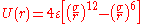Another example is the Born (ionic) model of the ionic lattice. The first term in the next equation is Coulomb's law
Coulomb's law
Coulomb's law or Coulomb's inverse-square law, is a law of physics describing the electrostatic interaction between electrically charged particles. It was first published in 1785 by French physicist Charles Augustin de Coulomb and was essential to the development of the theory of electromagnetism...

for a pair of ions, the second term is the short-range repulsion explained by Pauli's exclusion principle and the final term is the dispersion interaction term. Usually, a simulation only includes the dipolar term, although sometimes the quadrupolar term is included as well.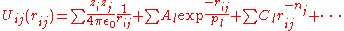In many-body potentials
N-body problem
The n-body problem is the problem of predicting the motion of a group of celestial objects that interact with each other gravitationally. Solving this problem has been motivated by the need to understand the motion of the Sun, planets and the visible stars...

, the potential energy includes the effects of three or more particles interacting with each other. In simulations with pairwise potentials, global interactions in the system also exist, but they occur only through pairwise terms. In many-body potentials, the potential energy cannot be found by a sum over pairs of atoms, as these interactions are calculated explicitly as a combination of higher-order terms. In the statistical view, the dependency between the variables cannot in general be expressed using only pairwise products of the degrees of freedom. For example, the Tersoff potential, which was originally used to simulate carbon
Carbon
Carbon is the chemical element with symbol C and atomic number 6. As a member of group 14 on the periodic table, it is nonmetallic and tetravalent—making four electrons available to form covalent chemical bonds...

, silicon
Silicon
Silicon is a chemical element with the symbol Si and atomic number 14. A tetravalent metalloid, it is less reactive than its chemical analog carbon, the nonmetal directly above it in the periodic table, but more reactive than germanium, the metalloid directly below it in the table...

and germanium
Germanium
Germanium is a chemical element with the symbol Ge and atomic number 32. It is a lustrous, hard, grayish-white metalloid in the carbon group, chemically similar to its group neighbors tin and silicon. The isolated element is a semiconductor, with an appearance most similar to elemental silicon....

and has since been used for a wide range of other materials, involves a sum over groups of three atoms, with the angles between the atoms being an important factor in the potential. Other examples are the embedded-atom method
Embedded atom model
In computational chemistry and computational physics, the embedded atom model, embedded-atom method or EAM, is an approximation describing the energy between two atoms. The energy is a function of a sum of functions of the separation between an atom and its neighbors. In the original model, by...

(EAM) and the Tight-Binding Second Moment Approximation (TBSMA) potentials, where the electron density of states in the region of an atom is calculated from a sum of contributions from surrounding atoms, and the potential energy contribution is then a function of this sum.

### Semi-empirical potentials

Semi-empirical
Semi-empirical quantum chemistry methods
Semi-empirical quantum chemistry methods are based on the Hartree-Fock formalism, but make many approximations and obtain some parameters from empirical data. They are very important in computational chemistry for treating large molecules where the full Hartree-Fock method without the...

potentials make use of the matrix representation from quantum mechanics. However, the values of the matrix elements are found through empirical formulae that estimate the degree of overlap of specific atomic orbitals. The matrix is then diagonalized to determine the occupancy of the different atomic orbitals, and empirical formulae are used once again to determine the energy contributions of the orbitals.

There are a wide variety of semi-empirical potentials, known as tight-binding
Tight binding (physics)
In solid-state physics, the tight binding model is an approach to the calculation of electronic band structure using an approximate set of wave functions based upon superposition of wave functions for isolated atoms located at each atomic site. The method is closely related to the LCAO method used...

potentials, which vary according to the atoms being modeled.

### Polarizable potentials

Most classical force fields implicitly include the effect of polarizability
Polarizability
Polarizability is the measure of the change in a molecule's electron distribution in response to an applied electric field, which can also be induced by electric interactions with solvents or ionic reagents. It is a property of matter...

, e.g. by scaling up the partial charges obtained from quantum chemical calculations. These partial charges are stationary with respect to the mass of the atom. But molecular dynamics simulations can explicitly model polarizability with the introduction of induced dipoles through different methods, such as Drude particle
Drude particle
Drude particles are model oscillators used to simulate the effects of electronic polarizability in the context of a classical molecular mechanics force field. It is based on the Drude model of mobile electrons...

s or fluctuating charges. This allows for a dynamic redistribution of charge between atoms which responds to the local chemical environment.

For many years, polarizable MD simulations have been touted as the next generation. For homogenous liquids such as water, increased accuracy has been achieved through the inclusion of polarizability. Some promising results have also been achieved for proteins. However, it is still uncertain how to best approximate polarizability in a simulation.

### Potentials in Ab-initio methods

In classical molecular dynamics, a single potential energy surface (usually the ground state) is represented in the force field. This is a consequence of the Born-Oppenheimer approximation
Born-Oppenheimer approximation
In quantum chemistry, the computation of the energy and wavefunction of an average-size molecule is a formidable task that is alleviated by the Born–Oppenheimer approximation, named after Max Born and J. Robert Oppenheimer. For instance the benzene molecule consists of 12 nuclei and 42...

. In excited states, chemical reactions or when a more accurate representation is needed, electronic behavior can be obtained from first principles by using a quantum mechanical method, such as Density Functional Theory
Density functional theory
Density functional theory is a quantum mechanical modelling method used in physics and chemistry to investigate the electronic structure of many-body systems, in particular atoms, molecules, and the condensed phases. With this theory, the properties of a many-electron system can be determined by...

. This is known as Ab Initio Molecular Dynamics (AIMD). Due to the cost of treating the electronic degrees of freedom, the computational cost of this simulations is much higher than classical molecular dynamics. This implies that AIMD is limited to smaller systems and shorter periods of time.

Ab-initio
Ab initio
ab initio is a Latin term used in English, meaning from the beginning.ab initio may also refer to:* Ab Initio , a leading ETL Tool Software Company in the field of Data Warehousing.* ab initio quantum chemistry methods...

quantum-mechanical
Quantum chemistry
Quantum chemistry is a branch of chemistry whose primary focus is the application of quantum mechanics in physical models and experiments of chemical systems...

methods may be used to calculate the potential energy
Potential energy surface
A potential energy surface is generally used within the adiabatic or Born–Oppenheimer approximation in quantum mechanics and statistical mechanics to model chemical reactions and interactions in simple chemical and physical systems...

of a system on the fly, as needed for conformations in a trajectory. This calculation is usually made in the close neighborhood of the reaction coordinate
Reaction coordinate
In chemistry, a reaction coordinate is an abstract one-dimensional coordinate which represents progress along a reaction pathway. It is usually a geometric parameter that changes during the conversion of one or more molecular entities....

. Although various approximations may be used, these are based on theoretical considerations, not on empirical fitting. Ab-Initio calculations produce a vast amount of information that is not available from empirical methods, such as density of electronic states or other electronic properties. A significant advantage of using ab-initio methods is the ability to study reactions that involve breaking or formation of covalent bonds, which correspond to multiple electronic states.

### Hybrid QM/MM

QM (quantum-mechanical) methods are very powerful. However, they are computationally expensive, while the MM (classical or molecular mechanics) methods are fast but suffer from several limitations (require extensive parameterization; energy estimates obtained are not very accurate; cannot be used to simulate reactions where covalent bonds are broken/formed; and are limited in their abilities for providing accurate details regarding the chemical environment). A new class of method has emerged that combines the good points of QM (accuracy) and MM (speed) calculations. These methods are known as mixed or hybrid quantum-mechanical and molecular mechanics methods (hybrid QM/MM).

The most important advantage of hybrid QM/MM methods is the speed. The cost of doing classical molecular dynamics (MM) in the most straightforward case scales O(n2), where n is the number of atoms in the system. This is mainly due to electrostatic interactions term (every particle interacts with every other particle). However, use of cutoff radius, periodic pair-list updates and more recently the variations of the particle-mesh Ewald's (PME) method has reduced this between O(n) to O(n2). In other words, if a system with twice as many atoms is simulated then it would take between two to four times as much computing power. On the other hand the simplest ab-initio calculations typically scale O(n3) or worse (Restricted Hartree-Fock
Hartree-Fock
In computational physics and chemistry, the Hartree–Fock method is an approximate method for the determination of the ground-state wave function and ground-state energy of a quantum many-body system....

calculations have been suggested to scale ~O(n2.7)). To overcome the limitation, a small part of the system is treated quantum-mechanically (typically active-site of an enzyme) and the remaining system is treated classically.

In more sophisticated implementations, QM/MM methods exist to treat both light nuclei susceptible to quantum effects (such as hydrogens) and electronic states. This allows generation of hydrogen wave-functions (similar to electronic wave-functions). This methodology has been useful in investigating phenomena such as hydrogen tunneling. One example where QM/MM methods have provided new discoveries is the calculation of hydride transfer in the enzyme liver alcohol dehydrogenase
Alcohol dehydrogenase
Alcohol dehydrogenases are a group of dehydrogenase enzymes that occur in many organisms and facilitate the interconversion between alcohols and aldehydes or ketones with the reduction of nicotinamide adenine dinucleotide...

. In this case, tunneling is important for the hydrogen, as it determines the reaction rate.

### Coarse-graining and reduced representations

At the other end of the detail scale are coarse-grained and lattice models. Instead of explicitly representing every atom of the system, one uses "pseudo-atoms" to represent groups of atoms. MD simulations on very large systems may require such large computer resources that they cannot easily be studied by traditional all-atom methods. Similarly, simulations of processes on long timescales (beyond about 1 microsecond) are prohibitively expensive, because they require so many timesteps. In these cases, one can sometimes tackle the problem by using reduced representations, which are also called coarse-grained models.

Examples for coarse graining (CG) methods are discontinuous molecular dynamics (CG-DMD) and Go-models. Coarse-graining is done sometimes taking larger pseudo-atoms. Such united atom approximations have been used in MD simulations of biological membranes. The aliphatic tails of lipids are represented by a few pseudo-atoms by gathering 2 to 4 methylene groups into each pseudo-atom.

The parameterization of these very coarse-grained models must be done empirically, by matching the behavior of the model to appropriate experimental data or all-atom simulations. Ideally, these parameters should account for both enthalpic
Enthalpy
Enthalpy is a measure of the total energy of a thermodynamic system. It includes the internal energy, which is the energy required to create a system, and the amount of energy required to make room for it by displacing its environment and establishing its volume and pressure.Enthalpy is a...

and entropic
Entropy
Entropy is a thermodynamic property that can be used to determine the energy available for useful work in a thermodynamic process, such as in energy conversion devices, engines, or machines. Such devices can only be driven by convertible energy, and have a theoretical maximum efficiency when...

contributions to free energy in an implicit way. When coarse-graining is done at higher levels, the accuracy of the dynamic description may be less reliable. But very coarse-grained models have been used successfully to examine a wide range of questions in structural biology, liquid crystal organization, and polymer glasses.

Examples of applications of coarse-graining:
• protein folding
Protein folding
Protein folding is the process by which a protein structure assumes its functional shape or conformation. It is the physical process by which a polypeptide folds into its characteristic and functional three-dimensional structure from random coil....

studies are often carried out using a single (or a few) pseudo-atoms per amino acid;
• liquid crystal
Liquid crystal
Liquid crystals are a state of matter that have properties between those of a conventional liquid and those of a solid crystal. For instance, an LC may flow like a liquid, but its molecules may be oriented in a crystal-like way. There are many different types of LC phases, which can be...

phase transitions have been examined in confined geometries and/or during flow using the Gay-Berne potential which describes anisotropic species;
• Polymer
Polymer
A polymer is a large molecule composed of repeating structural units. These subunits are typically connected by covalent chemical bonds...

glasses during deformation have been studied using simple harmonic or FENE
FENE
FENE stands for the finitely extensible nonlinear elastic model of a long-chained polymer. It simplifies the chain of monomers by connecting a sequence of beads with nonlinear springs...

springs to connect spheres described by the Lennard-Jones potential
Lennard-Jones potential
The Lennard-Jones potential is a mathematically simple model that approximates the interaction between a pair of neutral atoms or molecules. A form of the potential was first proposed in 1924 by John Lennard-Jones...

;
• DNA supercoiling has been investigated using 1-3 pseudo-atoms per basepair, and at even lower resolution;
• Packaging of double-helical DNA
DNA
Deoxyribonucleic acid is a nucleic acid that contains the genetic instructions used in the development and functioning of all known living organisms . The DNA segments that carry this genetic information are called genes, but other DNA sequences have structural purposes, or are involved in...

into bacteriophage
Bacteriophage
A bacteriophage is any one of a number of viruses that infect bacteria. They do this by injecting genetic material, which they carry enclosed in an outer protein capsid...

has been investigated with models where one pseudo-atom represents one turn (about 10 basepairs) of the double helix;
• RNA structure in the ribosome
Ribosome
A ribosome is a component of cells that assembles the twenty specific amino acid molecules to form the particular protein molecule determined by the nucleotide sequence of an RNA molecule....

and other large systems has been modeled with one pseudo-atom per nucleotide.

The simplest form of coarse-graining is the "united atom" (sometimes called "extended atom") and was used in most early MD simulations of proteins, lipids and nucleic acids. For example, instead of treating all four atoms of a CH3 methyl group explicitly (or all three atoms of CH2 methylene group), one represents the whole group with a single pseudo-atom. This pseudo-atom must, of course, be properly parameterized so that its van der Waals interactions with other groups have the proper distance-dependence. Similar considerations apply to the bonds, angles, and torsions in which the pseudo-atom participates. In this kind of united atom representation, one typically eliminates all explicit hydrogen atoms except those that have the capability to participate in hydrogen bonds ("polar hydrogens"). An example of this is the Charmm 19
CHARMM
CHARMM is the name of a widely used set of force fields for molecular dynamics as well as the name for the molecular dynamics simulation and analysis package associated with them...

force-field.

The polar hydrogens are usually retained in the model, because proper treatment of hydrogen bonds requires a reasonably accurate description of the directionality and the electrostatic interactions between the donor and acceptor groups. A hydroxyl group, for example, can be both a hydrogen bond donor and a hydrogen bond acceptor, and it would be impossible to treat this with a single OH pseudo-atom. Note that about half the atoms in a protein or nucleic acid are nonpolar hydrogens, so the use of united atoms can provide a substantial savings in computer time.

## Examples of applications

Molecular dynamics is used in many fields of science.
• First macromolecular MD simulation published (1977, Size: 500 atoms, Simulation Time: 9.2 ps=0.0092 ns, Program: CHARMM
CHARMM
CHARMM is the name of a widely used set of force fields for molecular dynamics as well as the name for the molecular dynamics simulation and analysis package associated with them...

precursor) Protein: Bovine Pancreatic Trypsine Inhibitor. This is one of the best studied proteins in terms of folding and kinetics. Its simulation published in Nature magazine paved the way for understanding protein motion as essential in function and not just accessory.

• MD is the standard method to treat collision cascade
A collision cascade is a set of nearby adjacent energetic collisions of atoms induced by an energetic particle in a solid or liquid....

s in the heat spike regime, i.e. the effects that energetic neutron
Neutron
The neutron is a subatomic hadron particle which has the symbol or , no net electric charge and a mass slightly larger than that of a proton. With the exception of hydrogen, nuclei of atoms consist of protons and neutrons, which are therefore collectively referred to as nucleons. The number of...

and ion irradiation have on solids an solid surfaces.

• MD simulations were successfully applied to predict the molecular basis of the most common protein mutation N370S, causing Gaucher Disease. In a follow-up publication it was shown that these blind predictions show a surprisingly high correlation with experimental work on the same mutant, published independently at a later point.

The following two biophysical examples are not run-of-the-mill MD simulations. They illustrate notable efforts to produce simulations of a system of very large size (a complete virus) and very long simulation times (500 microseconds):
• MD simulation of the complete satellite tobacco mosaic virus (STMV) (2006, Size: 1 million atoms, Simulation time: 50 ns, program: NAMD
NAMD
NAMD is a free-of-charge molecular dynamics simulation package written using the Charm++ parallel programming model, noted for its parallel efficiency and often used to simulate large systems...

) This virus is a small, icosahedral plant virus which worsens the symptoms of infection by Tobacco Mosaic Virus (TMV). Molecular dynamics simulations were used to probe the mechanisms of viral assembly. The entire STMV particle consists of 60 identical copies of a single protein that make up the viral capsid
Capsid
A capsid is the protein shell of a virus. It consists of several oligomeric structural subunits made of protein called protomers. The observable 3-dimensional morphological subunits, which may or may not correspond to individual proteins, are called capsomeres. The capsid encloses the genetic...

(coating), and a 1063 nucleotide single stranded RNA genome
Genome
In modern molecular biology and genetics, the genome is the entirety of an organism's hereditary information. It is encoded either in DNA or, for many types of virus, in RNA. The genome includes both the genes and the non-coding sequences of the DNA/RNA....

. One key finding is that the capsid is very unstable when there is no RNA inside. The simulation would take a single 2006 desktop computer around 35 years to complete. It was thus done in many processors in parallel with continuous communication between them.

• Folding Simulations of the Villin Headpiece
Villin
Villin is a 92.5 kDa tissue-specific actin-binding protein associated with the actin core bundle of the brush border. Villin contains multiple gelsolin-like domains capped by a small "headpiece" at the C-terminus consisting of a fast and independently-folding three-helix bundle that is stabilized...

in All-Atom Detail (2006, Size: 20,000 atoms; Simulation time: 500 µs = 500,000 ns, Program: Folding@home
Folding@home
Folding@home is a distributed computing project designed to use spare processing power on personal computers to perform simulations of disease-relevant protein folding and other molecular dynamics, and to improve on the methods of doing so...

) This simulation was run in 200,000 CPU's of participating personal computers around the world. These computers had the Folding@home program installed, a large-scale distributed computing effort coordinated by Vijay Pande at Stanford University. The kinetic properties of the Villin Headpiece protein were probed by using many independent, short trajectories run by CPU's without continuous real-time communication. One technique employed was the Pfold value analysis, which measures the probability of folding before unfolding of a specific starting conformation. Pfold gives information about transition state
Phi value analysis
Phi value analysis is an experimental protein engineering method used to study the structure of the folding transition state in small protein domains that fold in a two-state manner. Since the folding transition state is by definition a transient and partially unstructured state, its structure is...

structures and an ordering of conformations along the folding pathway
Protein folding
Protein folding is the process by which a protein structure assumes its functional shape or conformation. It is the physical process by which a polypeptide folds into its characteristic and functional three-dimensional structure from random coil....

. Each trajectory in a Pfold calculation can be relatively short, but many independent trajectories are needed.

### Integrators

• Verlet-Stoermer integration
• Runge-Kutta integration
• Beeman's algorithm
Beeman's algorithm
Beeman's algorithm is a method for numerically integrating ordinary differential equations of order 2, more specifically Newton's equations of motion \ddot x=A. It was designed to allow high numbers of particles in simulations of molecular dynamics. There is a direct or explicit and an implicit...

• Constraint algorithm
Constraint algorithm
In mechanics, a constraint algorithm is a method for satisfying constraints for bodies that obey Newton's equations of motion. There are three basic approaches to satisfying such constraints: choosing novel unconstrained coordinates , introducing explicit constraint forces, and minimizing...

s (for constrained systems)
• Symplectic integrator
Symplectic integrator
In mathematics, a symplectic integrator is a numerical integration scheme for a specific group of differential equations related to classical mechanics and symplectic geometry. Symplectic integrators form the subclass of geometric integrators which, by definition, are canonical transformations...

### Long-range interaction algorithms

• Ewald summation
Ewald summation
Ewald summation, named after Paul Peter Ewald, is a method for computing the interaction energies of periodic systems , particularly electrostatic energies. Ewald summation is a special case of the Poisson summation formula, replacing the summation of interaction energies in real space with an...

• Particle Mesh Ewald (PME)
• Particle-Particle Particle Mesh P3M
P3M
Particle-Particle-Particle Mesh is a Fourier-based Ewald summation method to calculate potentials in N-body simulations.The potential could be the electrostatic potential among N point charges i.e. molecular dynamics, the gravitational potential among N gas particles in e.g. smoothed particle...

### Parallelization strategies

• Domain decomposition method
Domain decomposition method
In mathematics, the additive Schwarz method, named after Hermann Schwarz, solves a boundary value problem for a partial differential equation approximately by splitting it into boundary value problems on smaller domains and adding the results.- Overview :...

(Distribution of system data for parallel computing
Parallel computing
Parallel computing is a form of computation in which many calculations are carried out simultaneously, operating on the principle that large problems can often be divided into smaller ones, which are then solved concurrently . There are several different forms of parallel computing: bit-level,...

)

## Related software

• Avizo
Avizo (software)
Avizo is a general-purpose commercial software application for scientific and industrial data visualization and analysis....

- 3d visualization and analysis software.
• BOSS
BOSS (molecular mechanics)
BOSS is a general-purpose molecular modeling program that performs molecular mechanics calculations, Metropolis Monte Carlo statistical mechanics simulations, and semiempirical AM1, PM3, and PDDG/PM3 quantum mechanics calculations...

- MC in OPLS
OPLS
The OPLS force field was developed by Prof. William L. Jorgensen at Purdue University and later at Yale University.-Functional form:The functional form of the OPLS force field is very similar to that of AMBER:...

• Chimera
UCSF Chimera
UCSF Chimera is an extensible program for interactive visualization and analysis of molecular structures and related data, including density maps, supramolecular assemblies, sequence alignments, docking results, trajectories, and conformational ensembles. High-quality and can be created...

- Molecular visualization and analysis package, including trajectory support.
• PyMol
PyMOL
PyMOL is an open-source, user-sponsored, molecular visualization system created by Warren Lyford DeLano and commercialized by DeLano Scientific LLC, which is a private software company dedicated to creating useful tools that become universally accessible to scientific and educational communities...

- Molecular Visualization software written in python.
• Sirius
Sirius visualization software
Sirius is a molecular modeling and analysis system developed at San Diego Supercomputer Center. Sirius is designed to support advanced user requirements that go beyond simple display of small molecules and proteins...

- Molecular modeling, analysis and visualization of MD trajectories.
• VMD
Visual Molecular Dynamics
- External links :* * *...

- MD simulation trajectories can be visualized and analyzed.
• Folding@Home - A distributed computing
Distributed computing
Distributed computing is a field of computer science that studies distributed systems. A distributed system consists of multiple autonomous computers that communicate through a computer network. The computers interact with each other in order to achieve a common goal...

project to study protein folding
Protein folding
Protein folding is the process by which a protein structure assumes its functional shape or conformation. It is the physical process by which a polypeptide folds into its characteristic and functional three-dimensional structure from random coil....

.

## Specialized hardware for MD simulations

• Anton
Anton (computer)
Anton is a massively parallel supercomputer designed and built by D. E. Shaw Research in New York. It is a special-purpose system for molecular dynamics simulations of proteins and other biological macromolecules...

- A specialized, massively parallel supercomputer designed to execute MD simulations.
• MDGRAPE - A special purpose system built for molecular dynamics simulations, especially protein structure prediction.

### General references

• M. P. Allen, D. J. Tildesley (1989) Computer simulation of liquids. Oxford University Press. ISBN 0-19-855645-4.
• J. A. McCammon, S. C. Harvey (1987) Dynamics of Proteins and Nucleic Acids. Cambridge University Press. ISBN 0521307503 (hardback).
• D. C. Rapaport (1996) The Art of Molecular Dynamics Simulation. ISBN 0-521-44561-2.
• J. M. Haile (2001) Molecular Dynamics Simulation: Elementary Methods. ISBN 0-471-18439-X
• R. J. Sadus, Molecular Simulation of Fluids: Theory, Algorithms and Object-Orientation, 2002, ISBN 0-444-51082-6
• Oren M. Becker, Alexander D. Mackerell Jr, Benoît Roux, Masakatsu Watanabe (2001) Computational Biochemistry and Biophysics. Marcel Dekker. ISBN 0-8247-0455-X.
• Andrew Leach (2001) Molecular Modelling: Principles and Applications. (2nd Edition) Prentice Hall. ISBN 978-0582382107.
• Tamar Schlick (2002) Molecular Modeling and Simulation. Springer. ISBN 0-387-95404-X.
• William Graham Hoover (1991) Computational Statistical Mechanics, Elsevier, ISBN 0-444-88192-1.
• D.J. Evans and G.P. Morriss (2008) Statistical Mechanics of Nonequilibrium Liquids, Second Edition, Cambridge University Press,ISBN 978-0-521-85791-8.